﻿ 水下航行体拖缆盘绕器阻力特性分析及优化设计
 舰船科学技术2020, Vol. 42Issue (8): 101-104    DOI: 10.3404/j.issn.1672-7649.2020.08.019PDF

Analysis resistance characteristics and optimum design of underwater vehicle cable winder
ZHANG La-ming, CAO Yao-ming, LIU Tian-jiao, ZHANG Yi-jun
Kunming Shipborne Equipment Research and Test Center, Kunming 650051, China
Abstract: In order to improve the installation reliability of the external towing cable and reduce the influence of the towing winder on the navigation attitude of the underwater vehicle, drag reduction of the towing winder is necessary during sailing. According to the requirement of drag reduction, the cross-section profile of the winder is optimized on the basis of its circular cross-section, aiming at minimizing the drag of the winder on the underwater vehicle. By using CFX software to simulate the drag of the winder during sailing, it is calculated that the maximum drag reduction of the symmetrical water droplet cross-section winder is 36.5% compared with the circular cross-section winder, and the drag reduction effect is obvious. The test results also show that the symmetrical water droplet cross-section winder has reliable locking and smooth unlocking, which verifies the simulation results.
Key words: towing cable     fluid resistance     optimization     FEM
0 引　言

1 圆形截面盘绕器有限元仿真 1.1 控制方程

 \left\{\begin{aligned} & \frac{\partial {u}_{i}}{\partial {x}_{i}}=0 {\text{，}}\\ & \frac{\partial {u}_{i}}{\partial t}+\frac{\partial }{\partial {x}_{j}}\left({u}_{i}{u}_{j}\right)=-\frac{1}{\rho }\frac{\partial p}{\partial {x}_{i}}+\frac{\partial }{\partial {x}_{j}}\left(v\frac{\partial {u}_{i}}{\partial {x}_{j}}\right)\;{\text{。}}\end{aligned}\right. (1)

 \left\{\begin{aligned} & \rho \frac{{\rm{d}}k}{{\rm{d}}t}+\rho \frac{\partial \left(k{u}_{i}\right)}{\partial {x}_{i}}=\frac{\partial }{\partial {x}_{j}}\left({T}_{k}\frac{\partial k}{\partial {x}_{j}}\right)+{G}_{k}-{Y}_{k}{\text{，}}\\ & \rho \frac{{\rm{d}}\omega }{{\rm{d}}t}+\rho \frac{\partial \left(\omega {u}_{i}\right)}{\partial {x}_{i}}=\frac{\partial }{\partial {x}_{j}}\left({T}_{\omega }\frac{\partial \omega }{\partial {x}_{j}}\right)+{G}_{\omega }-{Y}_{\omega }+{D}_{\omega }\;{\text{。}}\end{aligned}\right. (2)

1.2 几何模型与网格划分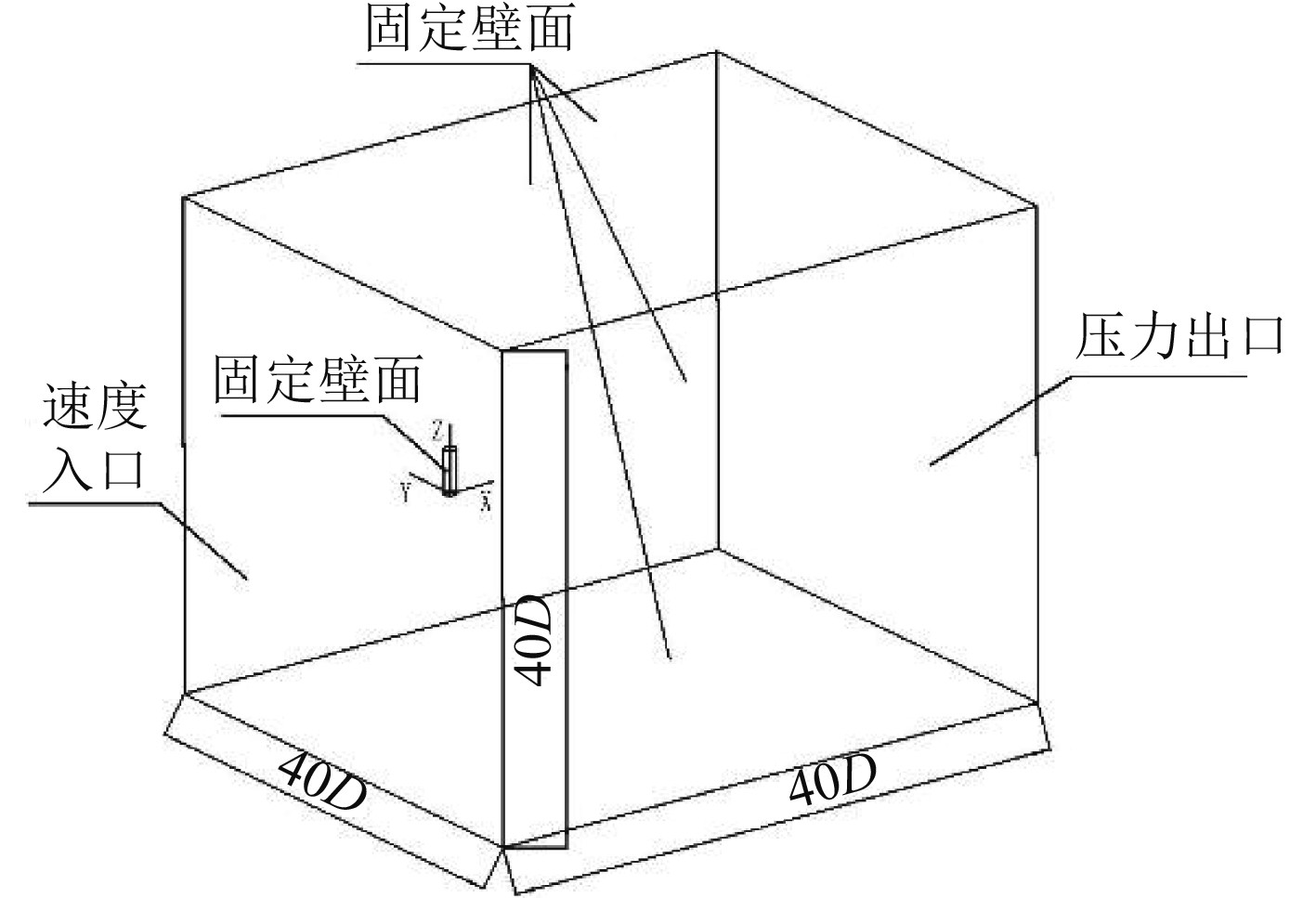图 1 计算域模型 Fig. 1 Model of computational domain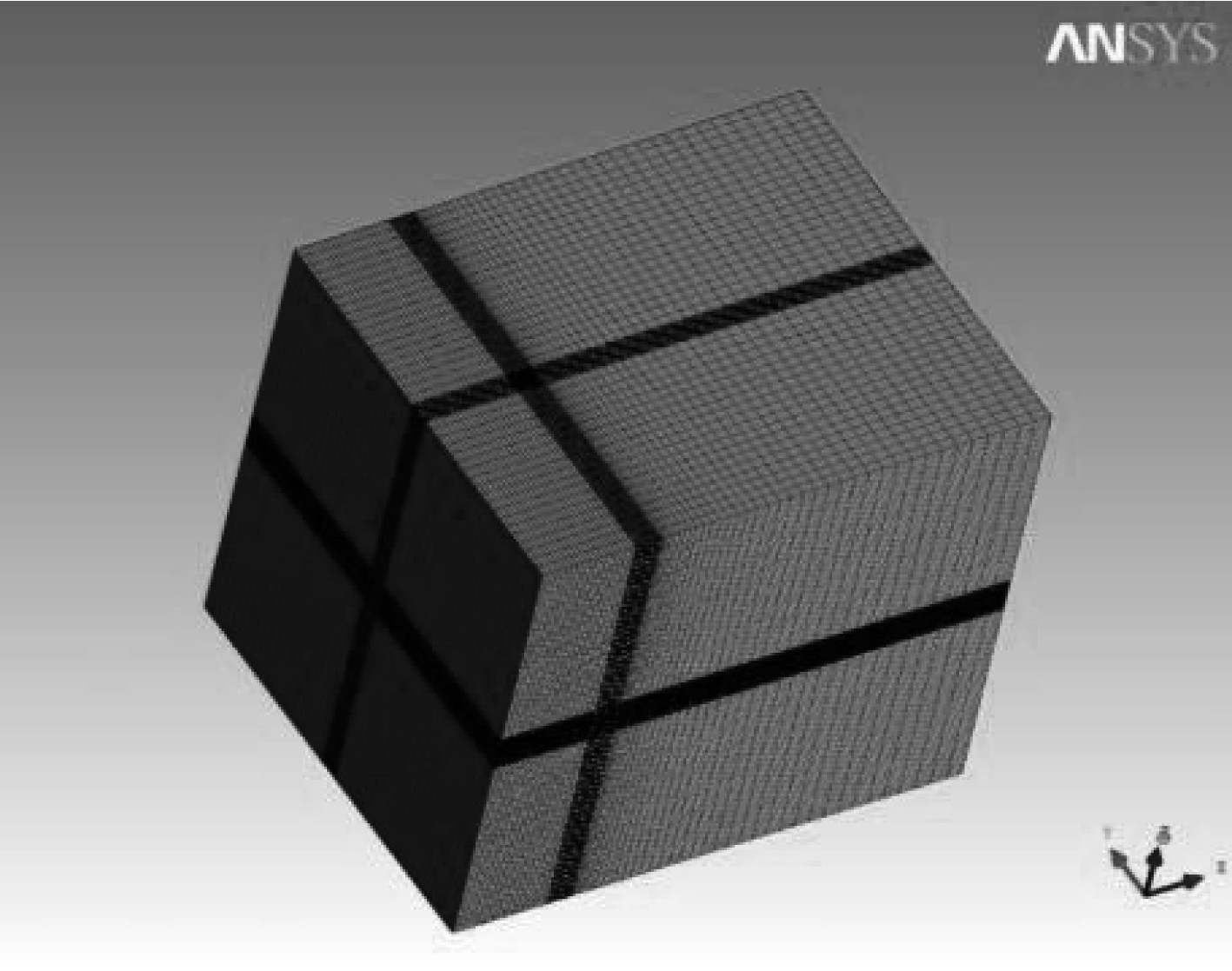图 2 盘绕器模型网格图 Fig. 2 Model grid of the winder
1.3 边界条件

1.4 模型验证

 ${R}_{e}=\frac{uD}{v}{\text{。}}$ (3)

 ${R}_{e}=\frac{2F}{\rho {u}^{2}DL}{\text{。}}$ (4)表 1 计算结果与文献实验结果及模拟结果对比 Tab.1 Comparison of simulation and experimental results provided by the literatures

1.5 按实际工况计算盘绕器阻力表 2 不同速度下的阻力值 Tab.2 Resistance values at different velocities
2 盘绕器优化设计 2.1 优化方法选择

2.2 卡克斯线型截面计算结果

 $Y=\frac{\left(X-f\right)\sqrt{LX-{X}^{2}}}{2k} \cdot D{\text{。}}$ (5)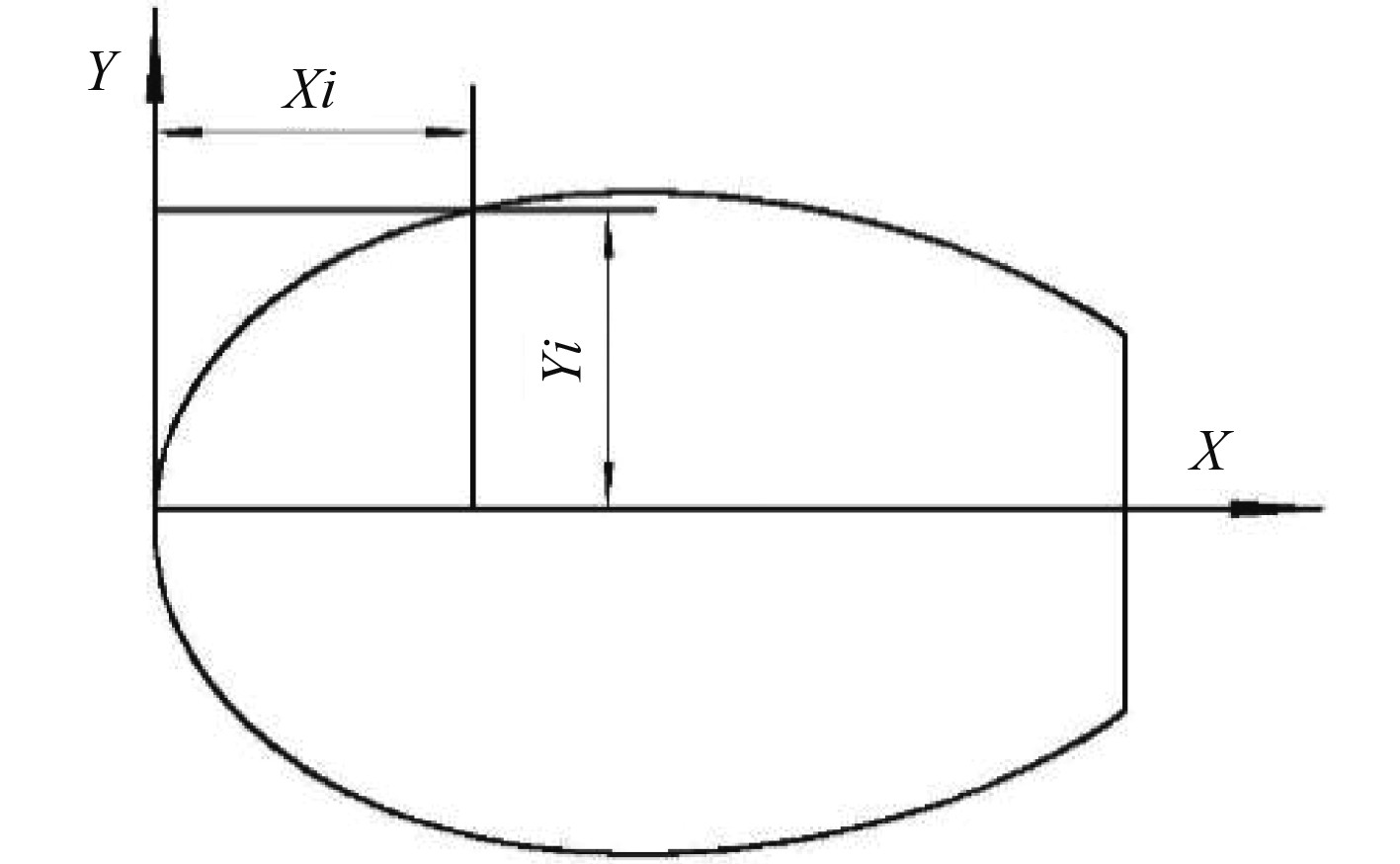图 3 卡克斯线型截面图 Fig. 3 Caksos linear cross section表 3 卡克斯线型盘绕器阻力值 Tab.3 Resistance values of Caksos linear winder
2.3 前后对称水滴线型截面计算结果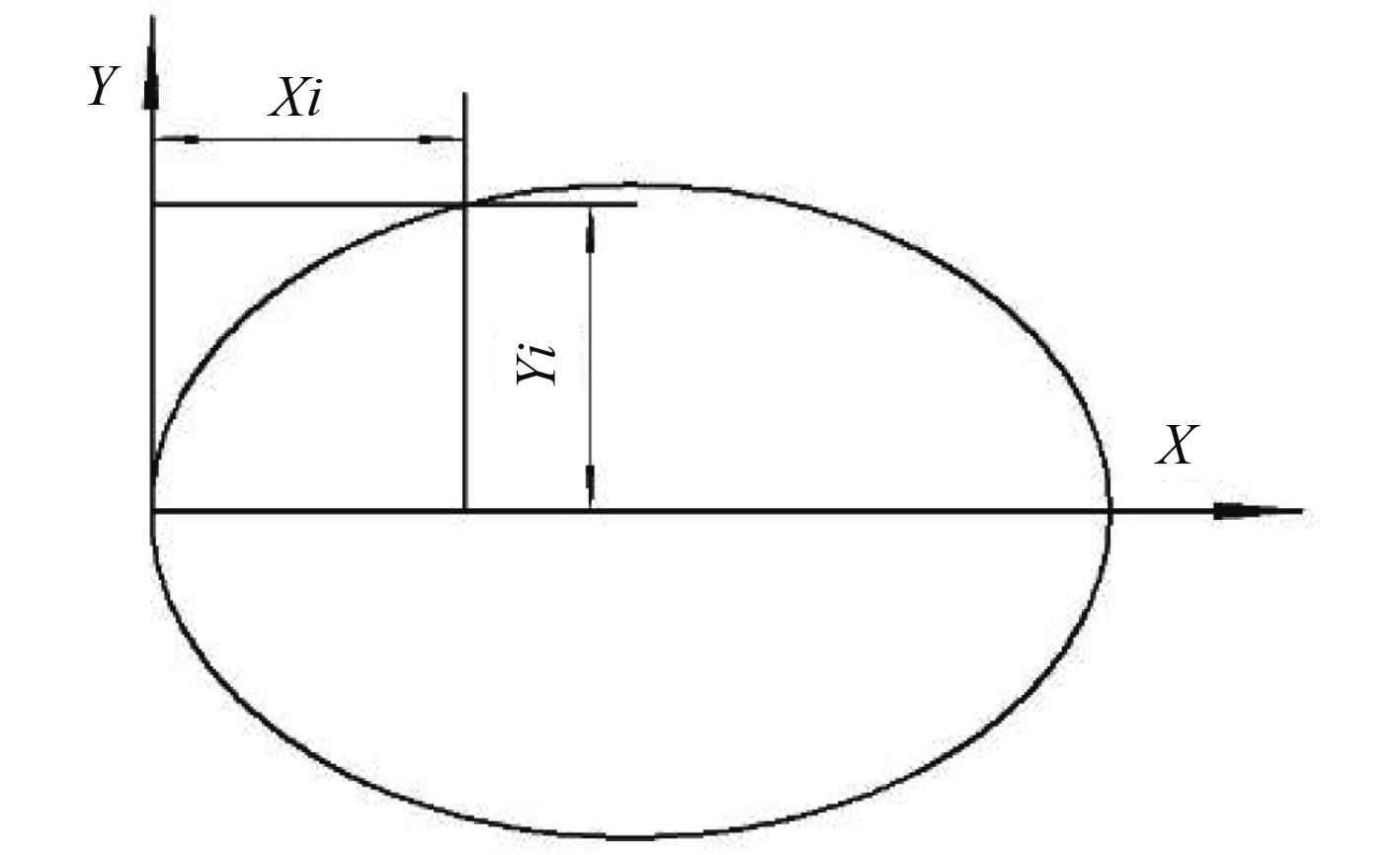图 4 前后对称水滴型截面图 Fig. 4 Symmetrical water droplet profile cross section
 $y=0.5D{\left[1-{\left(\frac{x}{{L}_{f}}\right)}^{{n}_{f}}\right]}^{\frac{1}{{n}_{f}}}\;{\text{。}}$ (6)表 4 前后对称水滴型截面盘绕器阻力值 Tab.4 Resistance values of symmetrical droplet linear winder
2.4 不同线型截面阻力计算结果对比表 5 各型截面盘绕器阻力值比较 Tab.5 Comparisons of resistance values of various cross section winder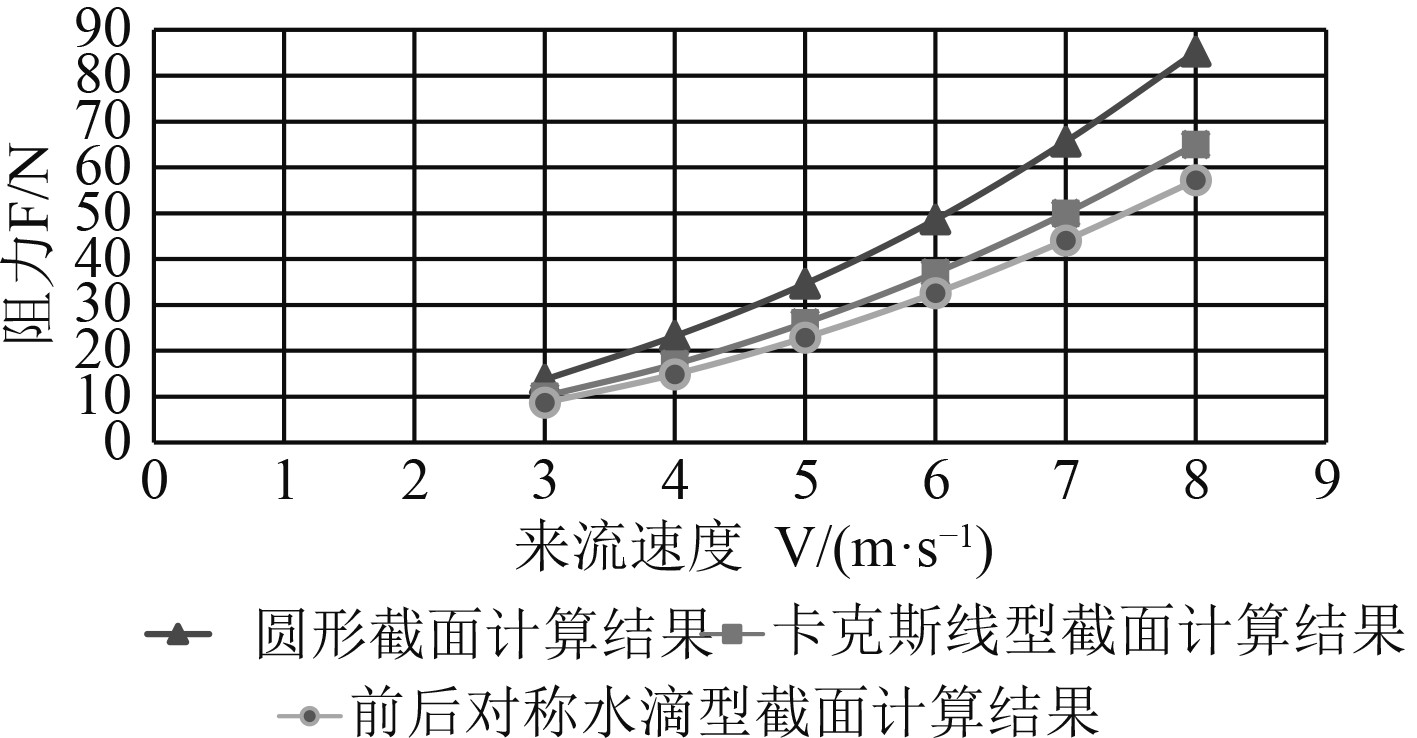图 5 不同线型截面盘绕器低速航行阻力值比较 Fig. 5 Comparisons of navigation resistance of winders with different linear sections

3 试验验证

4 结　语

1）从模拟计算结果可以看出，前后对称水滴型截面盘绕器迎流阻力小于卡克斯线型截面及圆形截面的盘绕器。相对圆形截面盘绕器，前后对称水滴型截面盘绕器最大可减阻36.5%，并且在试验过程中，前后对称水滴型截面盘绕器取得了较好的工程效果。

2）通过借用已有的线型，对水中航行物进行外形优化，可以降低航行物的迎流阻力，从而为快速进行工程设计提供了一种可行的设计方法。

  孔祥鑫. 多因素影响下柱体绕流及其涡激振动特性研究[D]. 青岛: 青岛科技大学, 2018.  沈立龙, 刘明维, 吴林键, 等. 亚临界雷诺数下圆柱和方柱绕流数值模拟[J]. 水道港口, 2014, 35(3): 227-233. DOI:10.3969/j.issn.1005-8443.2014.03.008  乔永亮, 桂洪斌, 刘祥鑫. 三维圆柱绕流数值模拟湍流方法的选择[J]. 水利水运工程学部, 2016(3): 119-125.  WORNOM S, OUVRARD H, SALVETTI M V, et al. Variational multiscale large-eddy simulations of the flow past a circular cylinder: Reynolds number effects[J]. Computers & Fluids, 2011, 47(1): 44-50.  王晓聪, 桂洪斌, 刘洋. 三维有限长圆柱绕流数值模拟[J]. 中国舰船研究, 2018, 13(2): 27-34. DOI:10.3969/j.issn.1673-3185.2018.02.004  秦丽萍. 水下航行体主体外形低阻低噪综合优化设计[D]. 西安: 西北工业大学, 2018.  杜月中, 闵健, 郭字洲. 流线型回转体外形设计综述与线型拟合[J]. 声学技术, 2004, 23(2): 93-97. DOI:10.3969/j.issn.1000-3630.2004.02.005  刘显龙. 水下拖曳体外形设计及其水动力性能分析[D]. 大连: 大连理工大学, 2010.